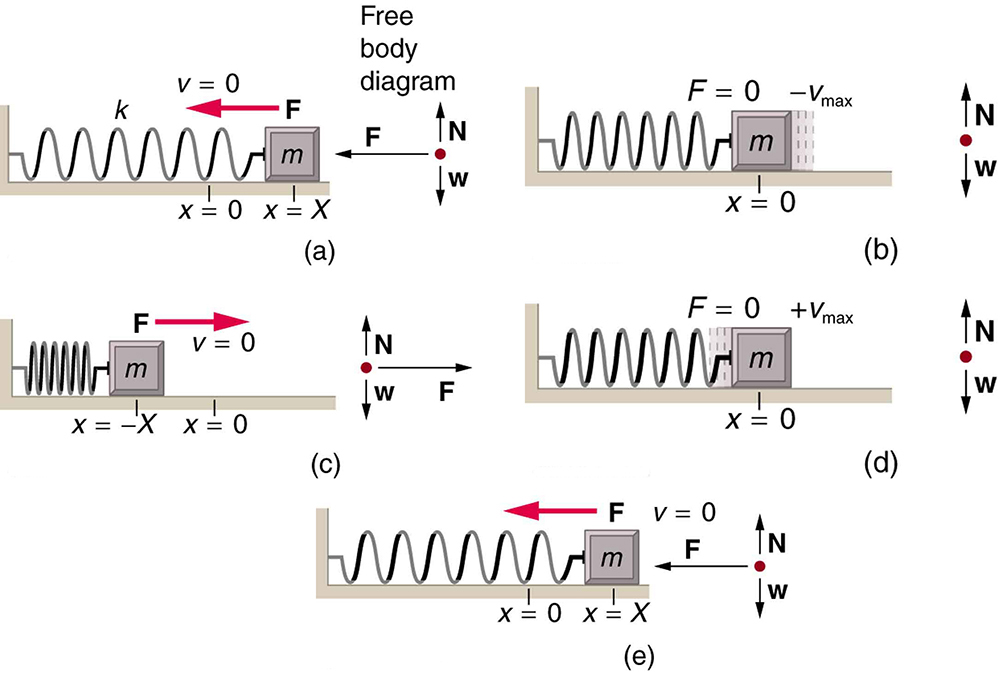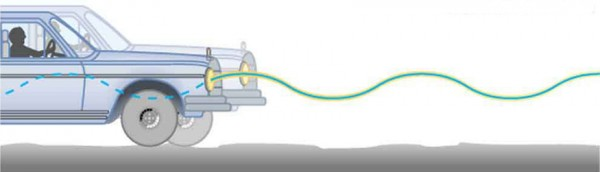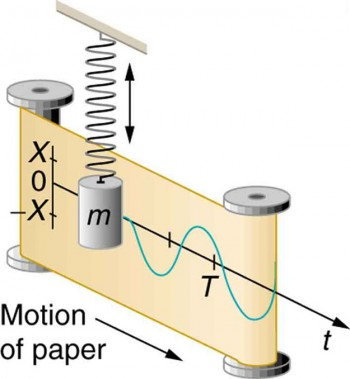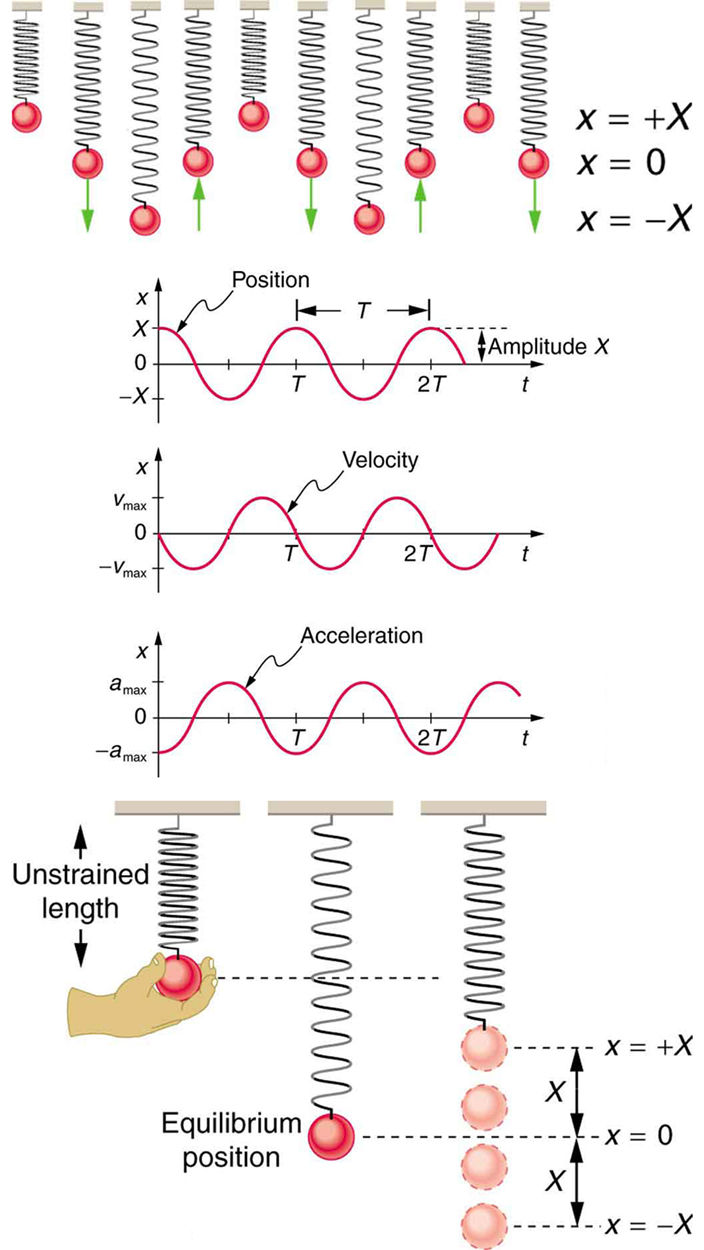## Simple Harmonic Motion: A Special Periodic Motion

### Learning Objectives

By the end of this section, you will be able to:

• Describe a simple harmonic oscillator.
• Explain the link between simple harmonic motion and waves.

The oscillations of a system in which the net force can be described by Hooke’s law are of special importance, because they are very common. They are also the simplest oscillatory systems. Simple Harmonic Motion (SHM) is the name given to oscillatory motion for a system where the net force can be described by Hooke’s law, and such a system is called a simple harmonic oscillator. If the net force can be described by Hooke’s law and there is no damping (by friction or other non-conservative forces), then a simple harmonic oscillator will oscillate with equal displacement on either side of the equilibrium position, as shown for an object on a spring in Figure 1. The maximum displacement from equilibrium is called the amplitude X. The units for amplitude and displacement are the same, but depend on the type of oscillation. For the object on the spring, the units of amplitude and displacement are meters; whereas for sound oscillations, they have units of pressure (and other types of oscillations have yet other units). Because amplitude is the maximum displacement, it is related to the energy in the oscillation.Figure 1. An object attached to a spring sliding on a frictionless surface is an uncomplicated simple harmonic oscillator. When displaced from equilibrium, the object performs simple harmonic motion that has an amplitude X and a period T. The object’s maximum speed occurs as it passes through equilibrium. The stiffer the spring is, the smaller the period T. The greater the mass of the object is, the greater the period T.

### Take-Home Experiment: SHM and the Marble

Find a bowl or basin that is shaped like a hemisphere on the inside. Place a marble inside the bowl and tilt the bowl periodically so the marble rolls from the bottom of the bowl to equally high points on the sides of the bowl. Get a feel for the force required to maintain this periodic motion. What is the restoring force and what role does the force you apply play in the simple harmonic motion (SHM) of the marble?

What is so significant about simple harmonic motion? One special thing is that the period T and frequency f of a simple harmonic oscillator are independent of amplitude. The string of a guitar, for example, will oscillate with the same frequency whether plucked gently or hard. Because the period is constant, a simple harmonic oscillator can be used as a clock.

Two important factors do affect the period of a simple harmonic oscillator. The period is related to how stiff the system is. A very stiff object has a large force constant k, which causes the system to have a smaller period. For example, you can adjust a diving board’s stiffness—the stiffer it is, the faster it vibrates, and the shorter its period. Period also depends on the mass of the oscillating system. The more massive the system is, the longer the period. For example, a heavy person on a diving board bounces up and down more slowly than a light one.

In fact, the mass m and the force constant k are the only factors that affect the period and frequency of simple harmonic motion.

### Period of Simple Harmonic Oscillator

The period of a simple harmonic oscillator is given by

$T=2\pi\sqrt{\frac{m}{k}}\\$

and, because $f=\frac{1}{T}\\$, the frequency of a simple harmonic oscillator is

$f=\frac{1}{2\pi}\sqrt{\frac{k}{m}}\\$.

Note that neither T nor f has any dependence on amplitude.

### Take-Home Experiment: Mass and Ruler Oscillations

Find two identical wooden or plastic rulers. Tape one end of each ruler firmly to the edge of a table so that the length of each ruler that protrudes from the table is the same. On the free end of one ruler tape a heavy object such as a few large coins. Pluck the ends of the rulers at the same time and observe which one undergoes more cycles in a time period, and measure the period of oscillation of each of the rulers.

### Example 1. Calculate the Frequency and Period of Oscillations: Bad Shock Absorbers in a Car

If the shock absorbers in a car go bad, then the car will oscillate at the least provocation, such as when going over bumps in the road and after stopping (See Figure 2). Calculate the frequency and period of these oscillations for such a car if the car’s mass (including its load) is 900 kg and the force constant (k) of the suspension system is 6.53 × 104 N/m.Figure 2. The bouncing car makes a wavelike motion. If the restoring force in the suspension system can be described only by Hooke’s law, then the wave is a sine function. (The wave is the trace produced by the headlight as the car moves to the right.)

#### Strategy

The frequency of the car’s oscillations will be that of a simple harmonic oscillator as given in the equation $f=\frac{1}{2\pi}\sqrt{\frac{k}{m}}\\$. The mass and the force constant are both given.

#### Solution

Enter the known values of k and m:

$\displaystyle{f}=\frac{1}{2\pi}\sqrt{\frac{k}{m}}=\frac{1}{2\pi}\sqrt{\frac{6.53\times10^4\text{ N/m}}{900\text{ kg}}}\\$

Calculate the frequency:

$\frac{1}{2\pi}\sqrt{72.6/\text{s}^{-2}}=1.3656/\text{s}^{-1}\approx1.36/\text{s}^{-1}=1.36\text{ Hz}\\$

You could use $T=2\pi\sqrt{\frac{m}{k}}\\$ to calculate the period, but it is simpler to use the relationship $T=\frac{1}{f}\\$ and substitute the value just found for f:

$\displaystyle{T}=\frac{1}{f}=\frac{1}{1.356\text{ Hz}}=0.738\text{ s}\\$

#### Discussion

The values of T and f both seem about right for a bouncing car. You can observe these oscillations if you push down hard on the end of a car and let go.

## The Link between Simple Harmonic Motion and WavesFigure 3. The vertical position of an object bouncing on a spring is recorded on a strip of moving paper, leaving a sine wave.

If a time-exposure photograph of the bouncing car were taken as it drove by, the headlight would make a wavelike streak, as shown in Figure 2. Similarly, Figure 3 shows an object bouncing on a spring as it leaves a wavelike “trace of its position on a moving strip of paper. Both waves are sine functions. All simple harmonic motion is intimately related to sine and cosine waves.

The displacement as a function of time t in any simple harmonic motion—that is, one in which the net restoring force can be described by Hooke’s law, is given by

$x(t)=X\cos\frac{2\pi{t}}{T}\\$,

where X is amplitude. At = 0, the initial position is x0 = X, and the displacement oscillates back and forth with a period T. (When T, we get X again because cos 2π = 1.). Furthermore, from this expression for x, the velocity v as a function of time is given by

$v(t)=-v_{\text{max}}\sin\left(\frac{2\pi{t}}{T}\right)\\$, where $v_{\text{max}}=\frac{2\pi{X}}{T}=X\sqrt{\frac{k}{m}}\\$.

The object has zero velocity at maximum displacement—for example, v=0 when t=0, and at that time x=X. The minus sign in the first equation for v(t) gives the correct direction for the velocity. Just after the start of the motion, for instance, the velocity is negative because the system is moving back toward the equilibrium point. Finally, we can get an expression for acceleration using Newton’s second law. [Then we have x(t), v(t), t, and a(t), the quantities needed for kinematics and a description of simple harmonic motion.] According to Newton’s second law, the acceleration is $a=\frac{F}{m}=\frac{kx}{m}\\$. So, a(t) is also a cosine function:

$a(t)=-\frac{kX}{m}\cos\frac{2\pi{t}}{T}\\$.

Hence, a(t) is directly proportional to and in the opposite direction to a(t).

Figure 4 shows the simple harmonic motion of an object on a spring and presents graphs of x(t), v(t), and a(t) versus time.Figure 4. Graphs of and versus t for the motion of an object on a spring. The net force on the object can be described by Hooke’s law, and so the object undergoes simple harmonic motion. Note that the initial position has the vertical displacement at its maximum value X; v is initially zero and then negative as the object moves down; and the initial acceleration is negative, back toward the equilibrium position and becomes zero at that point.

The most important point here is that these equations are mathematically straightforward and are valid for all simple harmonic motion. They are very useful in visualizing waves associated with simple harmonic motion, including visualizing how waves add with one another.

#### Part 1

Suppose you pluck a banjo string. You hear a single note that starts out loud and slowly quiets over time. Describe what happens to the sound waves in terms of period, frequency and amplitude as the sound decreases in volume.

##### Solution

Frequency and period remain essentially unchanged. Only amplitude decreases as volume decreases.

#### Part 2

A babysitter is pushing a child on a swing. At the point where the swing reaches x, where would the corresponding point on a wave of this motion be located?

##### Solution

x is the maximum deformation, which corresponds to the amplitude of the wave. The point on the wave would either be at the very top or the very bottom of the curve.

## PhET Explorations: Masses and Springs

A realistic mass and spring laboratory. Hang masses from springs and adjust the spring stiffness and damping. You can even slow time. Transport the lab to different planets. A chart shows the kinetic, potential, and thermal energy for each spring.

## Selected Solutions

• Simple harmonic motion is oscillatory motion for a system that can be described only by Hooke’s law. Such a system is also called a simple harmonic oscillator.
• Maximum displacement is the amplitude X. The period T and frequency f of a simple harmonic oscillator are given by $T=2\pi\sqrt{\frac{m}{k}}\\$ and $f=\frac{1}{2\pi }\sqrt{\frac{k}{m}}\\$ , where m is the mass of the system.
• Displacement in simple harmonic motion as a function of time is given by $x\left(t\right)=X\text{cos}\frac{2\pi{t}}{T}\\$.
• The velocity is given by $v\left(t\right)=-{v}_{\text{max}}\text{sin}\frac{2\pi{t}}{T}\\$, where ${v}_{\text{max}}=\sqrt{\frac{k}{m}}X\\$.
• The acceleration is found to be $a(t)=-\frac{kX}{m}\cos\frac{2\pi{t}}{T}\\$.

### Conceptual Questions

1. What conditions must be met to produce simple harmonic motion?
2. (a) If frequency is not constant for some oscillation, can the oscillation be simple harmonic motion? (b) Can you think of any examples of harmonic motion where the frequency may depend on the amplitude?
3. Give an example of a simple harmonic oscillator, specifically noting how its frequency is independent of amplitude.
4. Explain why you expect an object made of a stiff material to vibrate at a higher frequency than a similar object made of a spongy material.
5. As you pass a freight truck with a trailer on a highway, you notice that its trailer is bouncing up and down slowly. Is it more likely that the trailer is heavily loaded or nearly empty? Explain your answer.
6. Some people modify cars to be much closer to the ground than when manufactured. Should they install stiffer springs? Explain your answer.

### Problems & Exercises

1. A type of cuckoo clock keeps time by having a mass bouncing on a spring, usually something cute like a cherub in a chair. What force constant is needed to produce a period of 0.500 s for a 0.0150-kg mass?
2. If the spring constant of a simple harmonic oscillator is doubled, by what factor will the mass of the system need to change in order for the frequency of the motion to remain the same?
3. A 0.500-kg mass suspended from a spring oscillates with a period of 1.50 s. How much mass must be added to the object to change the period to 2.00 s?
4. By how much leeway (both percentage and mass) would you have in the selection of the mass of the object in the previous problem if you did not wish the new period to be greater than 2.01 s or less than 1.99 s?
5. Suppose you attach the object with mass m to a vertical spring originally at rest, and let it bounce up and down. You release the object from rest at the spring’s original rest length. (a) Show that the spring exerts an upward force of 2.00 mg on the object at its lowest point. (b) If the spring has a force constant of 10.0 N/m and a 0.25-kg-mass object is set in motion as described, find the amplitude of the oscillations. (c) Find the maximum velocity.
6. A diver on a diving board is undergoing simple harmonic motion. Her mass is 55.0 kg and the period of her motion is 0.800 s. The next diver is a male whose period of simple harmonic oscillation is 1.05 s. What is his mass if the mass of the board is negligible?
7. Suppose a diving board with no one on it bounces up and down in a simple harmonic motion with a frequency of 4.00 Hz. The board has an effective mass of 10.0 kg. What is the frequency of the simple harmonic motion of a 75.0-kg diver on the board?
8. The device pictured in Figure 6 entertains infants while keeping them from wandering. The child bounces in a harness suspended from a door frame by a spring constant.Figure 6. This child’s toy relies on springs to keep infants entertained. (credit: By Humboldthead, Flickr)

(a) If the spring stretches 0.250 m while supporting an 8.0-kg child, what is its spring constant? (b) What is the time for one complete bounce of this child? (c) What is the child’s maximum velocity if the amplitude of her bounce is 0.200 m?

9. A 90.0-kg skydiver hanging from a parachute bounces up and down with a period of 1.50 s. What is the new period of oscillation when a second skydiver, whose mass is 60.0 kg, hangs from the legs of the first, as seen in Figure 7.Figure 7. The oscillations of one skydiver are about to be affected by a second skydiver. (credit: U.S. Army, www.army.mil)

## Glossary

amplitude: the maximum displacement from the equilibrium position of an object oscillating around the equilibrium position

simple harmonic motion: the oscillatory motion in a system where the net force can be described by Hooke’s law

simple harmonic oscillator: a device that implements Hooke’s law, such as a mass that is attached to a spring, with the other end of the spring being connected to a rigid support such as a wall

1. 2.37 N/m

3. 0.389 kg

6. 94.7 kg

9. 1.94 s